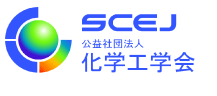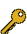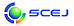English page## SY-69. \$B!Z%7%9%F%`!&>pJs!&%7%_%e%l!<%7%g%sIt2q%7%s%]%8%&%`![(B(2)\$B>pJsE}9g\$H%b%G%j%s%0%"%W%m!<%A(B

 \$B%*!<%,%J%\$%6!<(B: \$B?y;3(B \$B90OB!JEl5~Bg3X!K!&J?Hx(B \$B2mI'!JEl5~Bg3X!K(B

\$B%W%m%;%9;:6H\$K\$*\$\$\$F9gM}E*\$J0U;W7hDj\$r9T\$&\$?\$a\$K\$O!"MM!9\$JCN<1\$d>pJs\$NE}9gMxMQ\$,IT2D7g\$G\$"\$j!"\$=\$N4pHW\$H\$J\$k!V%b%G%k2=!W\$K4X\$9\$k8&5f\$r?<\$a\$kI,MW\$,\$"\$k!#K\%7%s%]%8%&%`\$G\$O!":rG/EY\$K0z\$-B3\$-!"2=3X!&0eLtIJ!&?)IJ;:6H\$d%j%5%\$%/%k;:6H!"\$5\$i\$K\$O]\$K!">pJsE}9g\$H\$=\$l\$r;Y1g\$9\$k%b%G%j%s%0%"%W%m!<%A\$NLr3d\$K\$D\$\$\$F9M\$(\$k!#(B
SIS\$BIt2q>^\$K\$D\$\$\$F\$O(B\$B\$3\$A\$i(B\$B\$r\$4Mw\$/\$@\$5\$\$!#(B

\$B:G=*99?7F|;~!'(B2016-11-29 11:54:01\$B\$3\$NJ,N`\$G\$h\$/;H\$o\$l(B
\$B\$F\$\$\$k%-!<%o!<%I(B
\$B%-!<%o!<%I(B\$B
risk evaluation1\$B7o(BAgriculture1\$B7o(BS-system method1\$B7o(BManagement Of Change1\$B7o(BData acquisition1\$B7o(Bplant lifecycle1\$B7o(BRenewable Energy1\$B7o(Bfood packaging1\$B7o(BCentralized and decentralized chemical processes1\$B7o(BRoute design by GIS1\$B7o(Bactivity model1\$B7o(BSteady-state sensitivity1\$B7o(BHydrous Ethanol1\$B7o(BInfrared spectroscopy1\$B7o(BQuality by Design1\$B7o(BSustainable society1\$B7o(Bequation-oriented process modelling1\$B7o(Bglobal system analysis1\$B7o(BProcess Systems Engineering1\$B7o(Bpharmaceutical manufacturing1\$B7o(BBiochemical systems theory1\$B7o(BSustainable Engineering1\$B7o(BCalibration-minimum approach1\$B7o(BBiomass1\$B7o(BProcess analytical technology1\$B7o(BHydrogen1\$B7o(BBig Data1\$B7o(Bsingle-use technology1\$B7o(BInternet of Things1\$B7o(Bplant alarm system1\$B7o(BDynamic vehicle load1\$B7o(Bbusiness process model1\$B7o(B\$B\$BHV9f(B\$B9V1iBjL\!?H/I=\$B%-!<%o!<%I(B\$BH/I=7A<0(B
37[\$BE8K>9V1i(B] IoT\$B\$H2=3X;:6H(B
(\$BG@9)Bg(B) (\$B@5(B)\$B;32<(B \$BA1G7(B
Internet of Things
Big Data
Process Systems Engineering
O
38[\$BE8K>9V1i(B] \$B?eAG%-%c%j%"@=B\$!&MxMQ%7%9%F%`3+H/\$K\$*\$1\$kF0E*%7%_%e%l!<%7%g%sE,MQ\$K4X\$9\$k9M;!(B
(\$B;:Am8&(B) (\$B@5(B)\$B!{>>K\(B \$B=(9T(B\$B!&(B \$B>.Eg(B \$B9(0l(B\$B!&(B (\$B@5(B)\$BG.3\$(B \$BNIJe(B\$B!&(B \$BVC(B \$B6(NO(B\$B!&(B \$BFqGH(B \$BE/:H(B\$B!&(B \$BDTB<(B \$BBs(B
Hydrogen
Renewable Energy
Dynamic Simulation
O
58\$BJQ994IM}\$NE,@5
(\$B2,;3>&Bg(B) (\$B@5(B)\$B!{L'NX(B \$B90;L(B\$B!&(B (\$B@EBg(B) (\$B@5(B)\$BIpED(B \$BOB9((B\$B!&(B (\$B0B1R8&(B) (\$B@5(B)\$BEgED(B \$B9T63(B\$B!&(B (\$BEl9)Bg(B) (\$B@5(B)\$B^
Sustainable Engineering
Management Of Change
O
64[\$BE8K>9V1i(B] \$B;}B32DG=\$J
(\$BElBg1!9)(B) (\$B@5(B)\$BJ?Hx(B \$B2mI'(B
Sustainable society
Trans-disciplinarily
Integration engineering
O
125\$BMF4oJqAu%i%\$%U%5%\$%/%k@_7W\$N%"%/%F%#%S%F%#%b%G%k(B
(\$BElBg1!9)(B) (\$B3X(B)\$B!{2#@n(B \$BD>5#(B\$B!&(B (\$B@5(B)\$B?y;3(B \$B90OB(B\$B!&(B (\$B@5(B)\$BJ?Hx(B \$B2mI'(B
food packaging
activity model
environmentally-conscious design
O
197\$BCm2A%b%G%k(B
(\$BElBg1!9)(B) (\$B3X(B)\$B!{GrH*(B \$B=UMh(B\$B!&(B (\$B@5(B)\$BJ?Hx(B \$B2mI'(B\$B!&(B (\$B@5(B)\$B?y;3(B \$B90OB(B
pharmaceutical manufacturing
risk evaluation
single-use technology
O
442\$BDj>o>uBV46EY7W;;%=%U%H(BiCOSMOS\$B\$N9b@-G=2=(B
(\$B6eBgG@(B) (\$B3X(B)\$B!{5\OF(B \$B29;R(B\$B!&(B (\$B@5(B)\$BGr@P(B \$BJ8=((B
Biochemical systems theory
S-system method
O
593\$BCO0h%(%M%k%.!<%7%9%F%`@_7W\$K\$*\$1\$kJ,;6E*>pJs\$NE}9gMxMQ(B
(\$BElBgAm3g%W%m(B) (\$B@5(B)\$B!{7s>>(B \$BM40lO:(B\$B!&(B (\$BElBg1!9)(B/\$BElBgAm3g%W%m(B) (\$B@5(B)\$BBg5WJ](B \$BC#Li(B\$B!&(B (\$BElBgAm3g%W%m(B/\$BElBg1!9)(B) (\$B@5(B)\$B5FCS(B \$B9/5*(B
Simulation
Data acquisition
Information modeling
O
614\$B%W%i%s%H%"%i!<%`%7%9%F%`%^%M%8%a%s%H\$+\$i8+\$?%W%i%s%H%i%\$%U%5%\$%/%k%(%s%8%K%"%j%s%0\$N6HL3%W%m%;%9%b%G%j%s%0%"%W%m!<%A(B
(\$B@EBg9)(B) (\$B@5(B)\$B!{IpED(B \$BOB9((B\$B!&(B (\$BL>9)Bg(B) (\$B@5(B)\$BIM8}(B \$B9';J(B\$B!&(B (\$B6eBg9)(B) (\$B@5(B)\$BLZB<(B \$BD>\$B!&(B (\$BJ!2,Bg(B) (\$B@5(B)\$BLnED(B \$B8-(B
plant alarm system
plant lifecycle
O
647\$B@V30%9%Z%/%H%k\$rMQ\$\$\$?%W%m%;%94F;k\$N\$?\$a\$NGHD9A*Br
(\$BElBg1!9)(B) (\$B3X(B)\$B!{\$B!&(B (\$B@5(B)\$B6b;R(B \$B90>;(B\$B!&(B (\$B@5(B)\$BA%DE(B \$B8x?M(B
Calibration-minimum approach
Infrared spectroscopy
Process analytical technology
O
677\$B%0%m!<%P%k%7%9%F%`%"%J%j%7%9\$K\$h\$k%W%m%;%9:GE,2=\$H%G%6%\$%s%9%Z!<%9@_7W(B
(\$B%T!<%(%9%\$!<%8%c%Q%s(B) (\$B@5(B)\$BGp20(B \$B<"(B
global system analysis
Quality by Design
equation-oriented process modelling
O
692\$BG@9)2#CG7?2r@O\$K4p\$E\$\$\$??"J*;q8;Mx3hMQ%7%9%F%`\$N6/2=\$H%b%G%j%s%0\$NLr3d(B
(\$BElBg1!9)(B) (\$B3X(B)\$B!{BgFbED(B \$B90B@O/(B\$B!&(B (\$BElBg1!9)(B/\$BElBgAm3g%W%m(B) (\$B@5(B)\$BBg5WJ](B \$BC#Li(B\$B!&(B (\$BElBgAm3g%W%m(B/\$BElBg1!9)(B) (\$B@5(B)\$B5FCS(B \$B9/5*(B
Biomass
Modeling
Agriculture
O
818\$B4^?e%(%?%N!<%k\$rMQ\$\$\$?%\$%*%s8r49
(\$BElKLBg(B) (\$B3X(B)\$B!{WE(B \$BR#NV(B\$B!&(B (\$B@5(B)\$BDD(B \$BW^pP(B\$B!&(B (\$B@5(B)\$BKL@n(B \$B>0H~(B\$B!&(B (\$B@5(B)\$BBgLn(B \$BH%(B\$B!&(B (\$B@5(B)\$BJ!Eg(B \$B9/M5(B
Life Cycle GHG Emission
FAEE
Hydrous Ethanol
O
862\$B2=3X%W%m%;%9\$NCO0hE83+J}<0\$N8!F\$(B:\$B8GBN
(\$BElKLBg(B) (\$B@5(B)\$B!{DD(B \$BW^pP(B\$B!&(B (\$B3X(B)\$BWE(B \$BR#NV(B\$B!&(B (\$B@5(B)\$BKL@n(B \$B>0H~(B\$B!&(B (\$B@5(B)\$BBgLn(B \$BH%(B\$B!&(B (\$B@5(B)\$BJ!Eg(B \$B9/M5(B
Centralized and decentralized chemical processes
Route design by GIS(C) 2016 \$B8x1W
E-mail: inquiry-48fwww3.scej.org
This page was generated byeasp 2.39; update.pl 2.37 (C)1999-2015 kawase